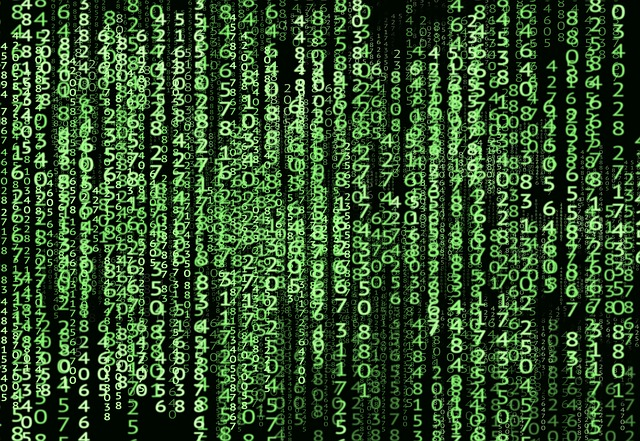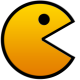# How Computers Represent Negative Binary Numbers?

Binary is not complicated. Once you learn how number systems work it’s pretty easy to go from decimal to binary, back, to add binary numbers, multiply them and so on (if you are not familiar with the binary system, check out this article on Wikipedia first).There’s one part of binary numbers that is not as striaght-forward, though, and that is the representation of negative binary numbers.

### Signed Magnitude

The simplest method to represent negative binary numbers is called Signed Magnitude: you use the leftmost digit as a sign indication, and treat the remaining bits as if they represented an unsigned integer. The convention is that if the leftmost digit (also called the most significant digit or most significant bit) is 0 the number is positive, if it’s 1 the number is negative. So:

``````00001010 = decimal 10
10001010 = decimal -10``````

That is why the range of positive numbers you can store in unsigned integers is larger than signed ones. For example, most computers use a 32-bit architecture these days, so integers will have 32 bits as well in C.

This means that an unsigned INT can go up to 4,294,967,296 (which is 2^32 – 1). You need to subtract one because the result of 2^32 starts from 1, while the first binary representation is 0.

Now if the INT is signed you won’t be able to use the leftmost bit. This means that your positive range will go up to 2,147,483,647 (which is 2^31 – 1). However you also have the negative values, and they go up to -2,147,483,647.

The main problem with this system is that it doesn’t support binary arithmetic (which is what the computer would naturally do). That is, if you add 10 and -10 binary you won’t get 0 as a result.

``````
00001010 (decimal 10)
+10001010 (decimal -10)
-------------
10010100 (decimal -20)``````

This doesn’t make much sense, and that’s why people came up with representations more suitable for a computer. Nonetheless there were some very early computers that used this system to represent negative numbers.

### One’s Complement

The One’s Complement of a binary number is basically another binary number which, when added to the original number, will make the result a binary number with 1s in all bits.

To obtain one’s complement you simply need to flip all the bits. Suppose we are working with unsigned integers.

Decimal 10 is represented as: 00001010

It’s one complement would be: 11110101

Notice that the complement is 245, which is 255 – 10. That is no co-incidence. The complement of a number (again, we talking unsigned) is the largest number represented with the number of bits available minus the number itself. Since we are using 8 bits here the maximum number represented is 255 (2^32 – 1). So the complement of 10 will be 245.

If we add the number and it’s complement the result should be 1s on all bits.

``````
00001010 (decimal 10)
+11110101 (decimal 245)
-------------
11111111 (decimal 255)``````

We could now say that the leftmost bit will indicate the signal of the number again. So 11110101 would be a negative number. What number? -10, because the complement of 11110101 is 00001010 (i.e., decimal 10).

Another example: suppose we want to represent -12 with the one’s complement system. First we need to represent 12 in binary, which is 00001100. Now we find it’s one’s complement, which is 11110011, and that is the -12.

As you can see, using the one’s complement system to represent negative numbers we would have two zeroes: 00000000 (could be seen as +0) and 11111111 (could be seen as -0).

Just as with the signed magnitude method, the range of numbers here goes from -2^(n-1) -1 to +2^(n-1) – 1, where n is the number of bits used to represent the numbers. If we had 8 bits the ranges would be from -127 up to 127.

With this representation the binary arithmetic problem is partially solved. If we add 12 and -12, for example, we’ll get -0 as the result, which makes sense.

``````
00001100 (decimal 12)
+11110011 (decimal -12)
-------------
11111111 (decimal -0)``````

I said this system partially solves the binary arithmetic problem because there are some special cases left.

For example, let’s add 3 with -1.

``````
00000011 (decimal 3)
+11111101 (decimal -2)
-------------
100000000 (decimal 256)
``````

But since we have only 8 bits to represent the numbers, the leftmost 1 will be discarded, and the result would be 00000000 (decimal +0).

This is not the answer we expected.

To fix the problem we just need to place the leftmost 1 (i.e., the carry) into the first bit.

``````
00000011 (decimal 3)
+11111101 (decimal -2)
-------------
00000000 (decimal +0)
+00000001 (the carry)
-------------
00000001 (decimal 1)
``````

Now it works. Let’s do one more example adding 10 and -5.

``````
00001010 (decimal 10)
+11111010 (decimal -5)
-------------
00000100 (decimal 4)
+00000001 (the carry)
-------------
00000101 (decimal 5)
``````

Works again!

This system was used by many computers at one point in time. For example, the PDP-1 (DEC’s first computer) used it.

### Two’s Complement

The Two’s Complement of a binary number is basically another number which, when added to the original, will make all bits become zeroes. You find a two’s complement by first finding the one’s complement, and then by adding 1 to it. If you think about it it makes perfect sense. The one’s complement, when added to the original number, will produce a binary number with 1s on all the bits. Add 1 to that and you’ll cause an overflow, setting every bit back to 0.

For example, let’s find the two’s complement of 12. The binary representation of 12 is 00001100. It’s one’s complement is 11110011. Add one to that and we have its two’s complement.

``````
11110011 (one's complement of 12)
+00000001 (decimal 1)
-------------
11110100 (two's complement of 12)
``````

Now if we add 12 with its two’s complement we should get all 0s.

``````
00001100 (decimal 12)
+11110100 (two's complement of 12)
-------------
00000000 (decimal 0)
``````

Once more we’ll use the most significant bit (i.e., the leftmost one) to represent the sign of the number. Let’s suppose we want to represent -5. First of find its one’s complement, which is 11111010, and then we add 1 to it. So -5 is represented as 11111011 in binary under the two’s complement system.

Now let’s add 12 with -5 to see if we’ll have the same problem that we had when using the one’s complement system:

``````
00001100 (decimal 12)
+11111011 (decimal -5)
-------------
00000111 (decimal 7)
``````

As you can see the result is correct, without the need to keep track/add the carry in case of overflow. Additionally, the number zero has a single representation now: 0000000.

This means that the two’s complement system pretty much solves all the binary arithmetic problems, and that is why it’s used by most computers these days.

If you have a negative binary number under the two’s complement system and want to convert it to you digital you simply remove 1 from it and then find its one’s complement.

Say we have this number in binary: 10010101

Removing one it becomes 10010100. Its one’s complement then is 01101011, which is 107 in decimal. So the original number represented -107.

As I mentioned before this method has only one representation for the zero, which is 00000000. 11111111 (which was also zero under the one’s complement system) will now be -1. And 10000000 will now be -128, meaning we gained one more number in the range.

That is, using the two’s complement system the range of numbers will go from -2^(n-1) up to +2^(n-1)-1. If we are using 8 bits this means that numbers will go from -128 up to 127.

Cool huh?

## 18 thoughts on “How Computers Represent Negative Binary Numbers?”

1.Edgar

I found your website thanks to daily blog tips.com keep up the good work.

2.Cmdline

Nice design. I like the white and green combo. Also the content is useful. The front page would look even better ith a picture for at least some post if not all posts.

Cmdlinetips.com

3.Craig

It is Binary. It is supposed to be boring

4.Cherryl Fennern

Very interesting info!Perfect just what I was searching for!

5.4autoinsurancequote

I absolutely love your blog and find many of your post’s to be what precisely I’m looking for. Do you offer guest writers to write content to suit your needs? I wouldn’t mind producing a post or elaborating on a few of the subjects you write with regards to here. Again, awesome site!

6.Star Wars

Very good explanation, Thanks .

7.partha

thank you..!! it was very helpful. I suppose there are a few typing errors, have a look ! thanks again

8.Anon

Very cool!

it is nicely written and it made the concepts pretty easy to understand.

Thank you!

9.Amar Agnihotri

I thimk there is one TYPO mistake in the range of one’s complement::
Just as with the signed magnitude method, the range of numbers here goes from -2^(n-1) -1 to +2^(n-1) – 1,
Just as with the signed magnitude method, the range of numbers here goes from -2^(n-1) +1 to +2^(n-1) – 1,

10.toni

Thank you so much. concepts explained in simple way

11.Mihir

Ecxellent explanation. Thank you very much!

12.Nagamani

Very interesting… clear explanation… After many years now I understood the negative numbers… Thank you…

13.Arvind

That was a fun read! One typo exists in “For example, let’s add 3 with -1.” where you proceed to subtract 2 from 3.

14.binarynab

Since we are using 8 bits here the maximum number represented is 255 (2^32 – 1).
Shouldn’t it be (2^8-1) ?.

15.Leo

Nice! But I also have a question. When I try to work out one’s complement of a signed negative number, does very bit flip except the significant bit?

16.dakshitha

Very well explained!

17.e

“Since we are using 8 bits here the maximum number represented is 255 (2^32 – 1)”

uhm?

18.e

the article is very clear, thank you